vol3-no1-4

Journal of Economics and Financial Analysis, Vol:3, No:1 (2019) 71-85

# A Modified Risk Parity Method for Asset Allocation

## Akhilesh MAEWAL*a, Joel R. BOCKb

a Yale University, United States

b University of California, San Diego, United States

## Abstract

We propose a return based modification of the portfolio variance matrix for asset allocation using risk parity. The modification is based upon a single scalar parameter which can be tuned to tailor the allocation for desired expected risk and/or return. The present work contributes a new twist on risk parity. While classical risk parity methods are based exclusively on volatility, the new solution (Modified Risk Parity ) considers both historical returns and their variance in the construction of an optimal, diversified investment portfolio. We present two examples for periods including the recent financial market crises. The results suggest that the modification may lead to significantly improved risk adjusted returns over those realized by the conventional risk parity method.

Keywords: Risk Parity; Asset Allocation; Decision Making; Portfolio Optimizaion.

JEL Classification: G11, G12, D81.

* Principal Scientist (Retd.), Honeywell Aerospace. E-mail address: amaewal@gmail.com (A. Maewal)

1. ## Introduction

Analytic construction of the asset distribution comprising an optimal invest- ment portfolio began with the work of Markowitz (1952), who describe an “efficient frontier” of expected return (E) and risk (V) for the portfolio. Markowitz showed that individual asset weights could be designed to maximize portfo- lio returns for a fixed accepted degree of risk, or alternatively, minimize risk given a constant level of expected portfolio return. The idea is to minimize risk exposure by diversifying its constituent asset classes, taking advantage of their respective uncorrelated temporal price dynamics.

The mean-variance solution has been criticized on the grounds that it is unstable with respect to small variations in inputs, thereby increasing variance of the expected return resulting in a sub-optimal solution (Merton, 1980; Michaud, 1989).

An alternative approach to risk reduction can be achieved by the so-called Risk Parity portfolios, considered by Qian (2005, 2006). In a risk parity portfolio, as originally formulated, the asset class distribution is allocated such that each instrument contributes an equal amount of risk to the overall portfolio. This effectively limits the potential loss contributed by any single asset class, and is expected to provide excellent returns for a constant level of risk. It is notable, however, that the general problem solved for asset allocation on the basis of risk parity can also be formulated as the classical Markowitz problem of minimization of the portfolio variance, albeit with a constraint that ensures that the weight of each component optimal portfolio be strictly positive (see Chaves et al. (2012), and also the Appendix below). From this perspective, risk parity is rather only a salient feature of a certain class of minimum variance portfolios, and it need not be invoked as an independent fundamental criterion for asset allocation.

Maillard et al. (2010) analyzed the theoretical unconstrained properties of risk parity portfolios. The authors assert that this methodology offers variance reduction lying somewhere between the heuristic methods of minimum variance (Haugen and Baker, 1996) and equally-weighted portfolios (Benartzi and Thaler, 2001). Each method has disadvantages to consider: the minimum variance approach may concentrate on a few asset classes with the lowest variance, while the 1/n portfolio ignores asset covariance and historical dispersion (Maillard et al., 2010).

Risk parity is an attractive philosophy in portfolio construction due to its intuitive appeal, straightforward computation (Chaves et al., 2012), and relatively strong performance in terms of risk-adjusted return when compared to other diver- sification strategies (e.g., see Clarke et al. (2012), Exhibit 2).

The present work contributes a new twist on risk parity. While classical risk parity methods are based exclusively on volatility, the new solution (Modified Risk Parity) considers both historical returns and their variance in the construction of an optimal, diversified investment portfolio1. In this sense, the variance-return relationships of the modified risk parity portfolios are evocative of Markowitz’ Efficient Frontier (see the figures below). Indi- viduals routinely invest in companies or sectors based on the expectation of performance in the next period. In Modified Risk Parity, this information is explicitly represented in the analytic construction of the portfolio, which simultaneously distributes risk across asset classes.

The degree to which historical returns influence the portfolio weights calculation is controlled by the magnitude of a single tuning parameter. The solution reduces to classical risk parity when the value of this parameter is set equal to zero.

Back-testing results for diversified portfolios spanning nearly two and a half decades are presented. These results demonstrate that, in certain scenarios, this method may lead to significant improvement on returns over those realized by the conventional risk parity method.

The primary application of this new method is low frequency re-balancing of long positions in a portfolio of negatively correlated financial instruments.

1 Although the proposed method can in principle be applied to more general cases, here we are interested only in annually updated portfolios with returns and their variance computed on the basis of the daily data for the year immediately prior to the beginning of the year of investment.

1. ## Analysis

We describe our method in the context of a portfolio which is rebalanced at the beginning of every year on the basis of the performance of the assets within it during the immediately prior year. Let r = [r1, r2, ...] be the vector of the annual returns of the assets of the prior year, and V the variance matrix of the daily returns of the prior year.

For a portfolio with two assets, or in the case that V is a diagonal matrix, the weights of the assets on the basis of the classical risk parity are given by: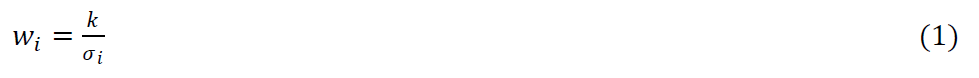where σi is the standard deviation of the daily returns of the asset i, given by √Vii and k is a constant determined by the condition ∑wi = 1.

In order to explicitly incorporate the asset returns in the methodology, we use return modifed standard deviations defined by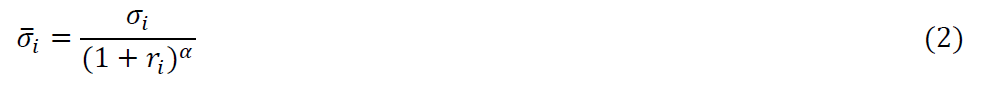where α, the return exponent, is a parameter to be specifed, and with the modifed weights determined exactly as in (1) above: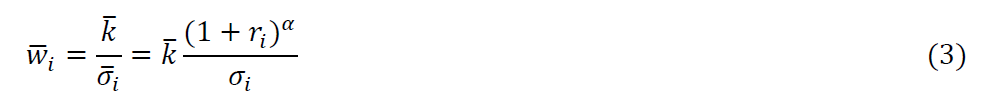The returns exponent α in (3) controls the extent to which the previous returns influence the allocation assignment looking forward. If α is positive, the allocation to assets with higher prior returns is higher relative to their weights as determined according to classical risk parity, reflecting the expectation that the assets whose returns were higher in the prior year will continue to outperform. Conversely, for a contra-momentum strategy, one specifies α to be negative, with the expectation that the assets with lower prior returns will perform better in the next year. Obviously, in the limiting case where α = 0, the allocation reduces to the classical risk parity solution. The solution regimes controlled by α can be summarized as follows:

α = 0 : Classical Risk Parity           (4)

α > 0 : Modified Risk Parity, Para-momentum

α < 0 : Modified Risk Parity, Contra-momentum

For the general case, the classical risk-parity weights for the vector w = [w1, w2, ...] are obtained by the solution of the problem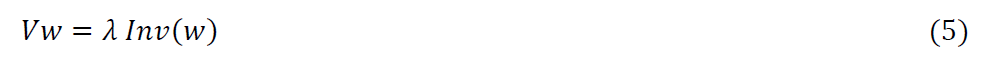subject to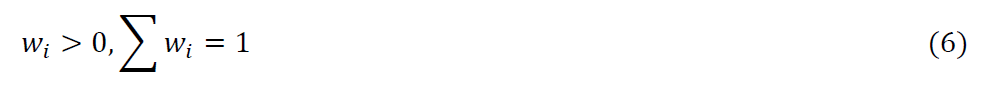Here, λ is a constant which is obtained as a part of the solution, and Inv(w) is the vector whose elements are the inverses of the corresponding elements of w, given by [1/w1, 1/w2,...].

It should be noted that although the problem in equations (5) and (6) is generally formulated as arising from the requirement that the risk contribution of each asset in the portfolio to the overall portfolio risk be the same (and hence the appellation risk parity) –an entirely novel concept at the time of its introduction– a more satisfactory way to view the problem is that its solution minimizes the portfolio variance, exactly as in the classical Markowitz approach, but subject to a constraint that enforces the requirement that the weight of each asset in the optimal portfolio be strictly positive (see Appendix). From this perspective, risk parity is just a property of a minimum variance portfolio, albeit designed with a constraint not used in the construction of the classical minimum variance portfolio, rather than an independent fundamental concept.

For the modified risk parity approach, we solve the same equation (5), except that the usual variance matrix V is replaced by the return modified variance matrix V, defined by.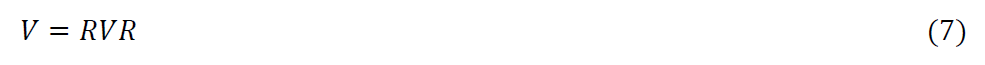where R is a diagonal matrix with Rii=1/(1+ri)2.

We should note that the modification proposed here retains the positive definiteness of the variance matrix, and allows the modified risk parity allocation problem to be solved by means of existing implementations of risk parity algorithms without any changes. Our own method is a Newton-type approach described in the following section.

3. ## A Newton-type method for Risk Parity Allocation

We have used, for the illustrative examples to follow, a Newton-type method for the solution of (5) and (6) that explicitly takes into account the positivity constraints on the asset weights. Another application of Newton’s method to risk parity based allocation without explicit imposition of the positivity constraint in the numerical algorithm has been described by Chaves et al. (2012).

To solve (5) with (6) for a portfolio containing N assets we use the following algorithm.

1. Choose a positive and small δ for the positivity constraints for w, the convergence parameter ϵ, and the damping parameter β, with 0 ≤ β < 1 and initialize.

1a. Calculate the initial solution w0 by ignoring the diagonal elements of V and (1), i.e.

2. Iterative solution for I = 0,1,2, ... till convergence

2a. Newton step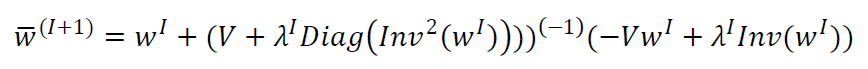where the elements of the vector Inv2(x) for any vector x are the squares of the elements of of Inv(x), and Diag(x) is the diagonal matrix containing the elements of the argument on its diagonal.

2b. Impose positivity constraints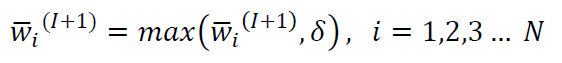2c. Normalize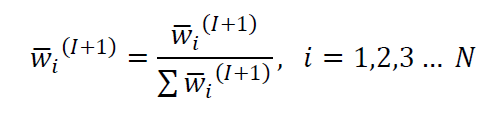2d. Damped update of w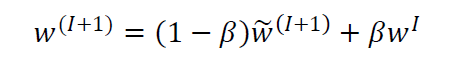2e. Update λ.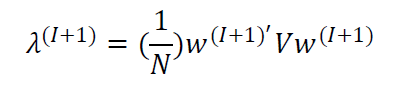2f. Test for convergence.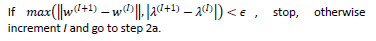The only free parameter in the modified risk parity method described above is the scalar that we call the return exponent- see equation (2) above. Some preliminary calculations for a period that included the recent financial crisis in 2008 and the dot-com implosion of the early 2000s suggested that this parameter –or more specifically, whether it is positive or negative– significantly affects the long term performance of modified risk parity portfolios. In other words, the decision on whether to use a para-momentum or a contra-momentum strategy for allocating the weights is of prime importance. We do not claim to have completely solved this problem, but a simple device appears to be quite effective for the examples that we use to illustrate the performance of modified risk parity portfolios.

Our approach rests on the assumption that the annual returns of equities relative to the annual returns of assets that are nominally negatively correlated to equities for a given year may be a good indicator of whether or not the high performers of the year will continue to outperform in the immediately following year. More concretely, for the construction of the portfolio for the year i, we consider the equity to bond return ratio series for the prior years.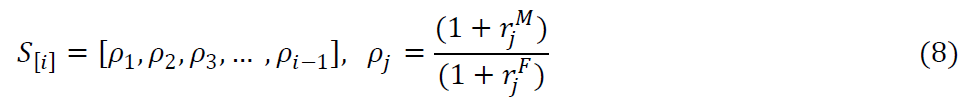where rMj is the annual return for the year j for equities and rFj is the annual return for the year j for bonds. In the examples shown below, we use the returns of a SP500 fund (Vanguard 500 Index Investor, VFINX) as a proxy for equity returns, and the returns of a long term treasury fund (Vanguard Long-Term Treasury Investment, VUSTX) as a proxy for bond returns.

We use the mean µS and the standard deviation σS of the array S[i] of the return ratios for the years prior to the year for which the portfolio is to be constructed and the return ratio for the prior year, ρi-1, to determine whether to use a contra-momentum (negative return exponent) strategy or a para- momentum strategy (positive return exponent) thus: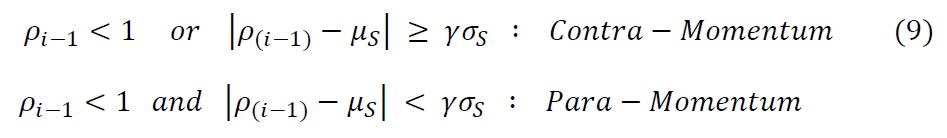where γ is a fixed constant (which we have specified to be 1.5 for all the examples described below). These conditions are based on the premise that normally the stock returns are higher than the bond returns and the equity to bond return ratio does not deviate too much from the mean of the historical return ratios.

The criterion in (9) is used for the Adaptive Modified Risk Parity (AMRP) calculations below to specify the sign of the return exponent for modifying the portfolio variance matrix for the modified risk parity calculations.

5. ## Summary of the procedure

6. As mentioned above, in the illustrative examples the portfolios are rebalanced at the beginning of every year on the basis of the historical annual and daily returns of the assets in the portfolio for the immediately prior year, with the latter used to compute the portfolio variance for input to the asset allocation calculations. The goal is to compare the performance of the portfolios constructed on the basis of the following variations of risk parity:

1. The classical risk parity procedure (RP).

2. The modified risk parity procedure (MRP) wherein the return expo- nent is fixed and positive for the entire period of back tests.

3. The (limited) adaptive modified risk parity procedure (AMRP) wherein the magnitude of the return exponent is fixed and identical for every year during the entire period of the back test, but its sign is adaptively varied according to (9) in order to allocate the weights appropriate for the estimated regime for the ensuing year. Clearly, we have not addressed here the interesting and the more general problem of how to specify both the sign and the magnitude of the return exponent on the basis of historical data available at the time of allocation.

Generally, in risk parity allocation, all of the assets in the basket are included in the calculations. In one of the examples below we also show some results for risk parity with a momentum overlay (RP/M, MRP/M and AMRP/M) that excludes one or more assets from the allocations on the basis of relative momentum.

1. ## Results

This section provides two examples of the practical application of the Modified Risk Parity method. Results of back-testing are compared against classical risk parity for diversified asset mixes covering periods that include both the dot-com bust of the early 2000s and the financial crisis of 2008.

1. ## Two Asset Example

Figures (1-4) display the performance metrics for the two asset basket of the SP500 ETF, SPY, and the long term treasury fund WHOSX for the magnitude of the return exponent alpha (see, Eq. (2)) in the range [0, 10] for the years 1995-2017. In this specific example, every variation of risk parity leads to substantial improvement in the risk based performance metrics (volatility, maximum drawdown, Sharpe ratio, and Sortino ratio) but only the adaptive modified risk parity method enhances the returns as well. We note here that due to the very low returns of some classical risk parity based portfolios, risk parity is often used in conjunction with leverage (see, e.g., Qian (2005)). The results for this example suggest that adaptive modified risk parity may obviate the need for such leverage.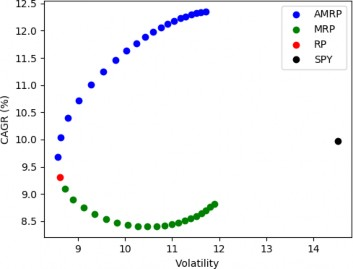Figure 1. Two Asset Example

Notes: RP is Classical Risk Parity; MRP is Modified Risk Parity; and AMRP is Adaptive Modified Risk Parity.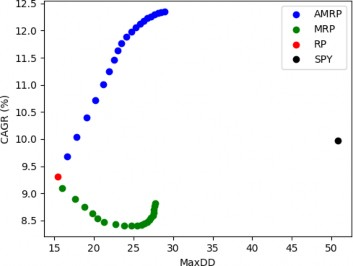Figure 2. Two Asset Example

Notes: RP is Classical Risk Parity; MRP is Modified Risk Parity; and AMRP is Adaptive Modified Risk Parity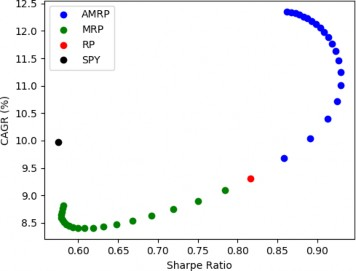Figure 3. Two Asset Example

Notes: RP is Classical Risk Parity; MRP is Modified Risk Parity; and AMRP is Adaptive Modified Risk Parity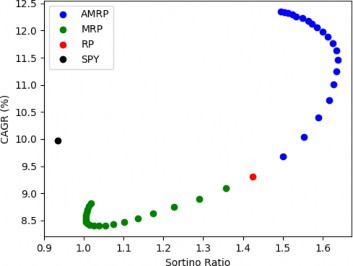Figure 4. Two Asset Example

Notes: RP is Classical Risk Parity; MRP is Modified Risk Parity; and AMRP is Adaptive Modified Risk Parity

2. ## A Multi-Asset Example

The basket for the muti-asset example consists of four index based equity ETFs: (i) SPY for the SP500 large cap stocks, (ii) MDY for mid-cap stocks, (iii) NAESX for small-cap stocks, and (iv) QQQ for the NASDAQ 100 index, and the long term treasury fund WHOSX. The results for the period 2000-2017 for the magnitude of the return exponent α is the range [0, 20] are displayed in Figures (5-8). The momentum overlay results in these plots (RP/M, MRP/M, and AMRP/M) were obtained by replacing, for a given year, one or or more of the equity ETFs whose returns for the prior year were lower than that of the treasury fund, by the treasury fund. Once again, the adaptive modified risk parity based portfolios, with and without the momentum overlay, yield significantly improved performance, both in terms of the risk based metrics as well as the total returns. Quite noteworthy is the substantially enhanced improvement in performance in the momentum portfolios using the modified risk parity approach.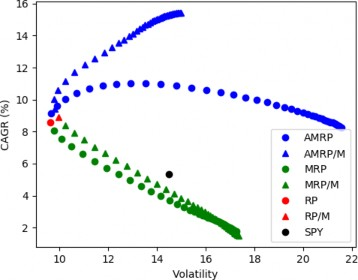Figure 5. Multi-Asset Example

Notes: RP is Classical Risk Parity; MRP is Modified Risk Parity; and AMRP is Adaptive Modified Risk Parity. The "/M" denotes results for the RP, MRP, and AMRP portfolios with a momentum overlay (see Section 3.2)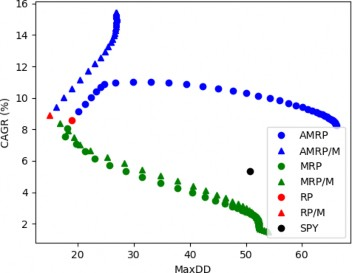Figure 6. Multi-Asset Example

Notes: RP is Classical Risk Parity; MRP is Modified Risk Parity; and AMRP is Adaptive Modified Risk Parity. The "/M" denotes results for the RP, MRP, and AMRP portfolios with a momentum overlay (see Section 3.2)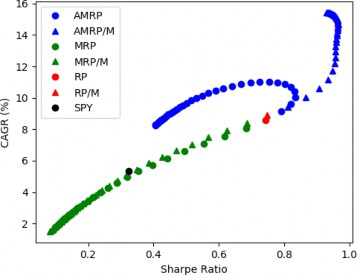Figure 7. Multi-Asset Example

Notes: RP is Classical Risk Parity; MRP is Modified Risk Parity; and AMRP is Adaptive Modified Risk Parity. The "/M" denotes results for the RP, MRP, and AMRP portfolios with a momentum overlay (see Section 3.2)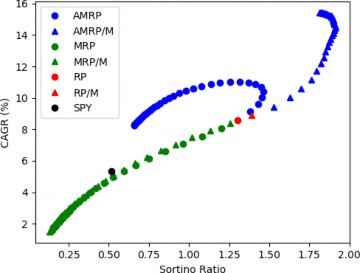Figure 8. Multi-Asset Example

Notes: RP is Classical Risk Parity; MRP is Modified Risk Parity; and AMRP is Adaptive Modified Risk Parity. The "/M" denotes results for the RP, MRP, and AMRP portfolios with a momentum overlay (see Section 3.2)

## 5. Concluding Remarks

The main contribution of our work is an adaptive modification of the portfolio variance matrix that can be used for incorporating asset returns for asset allocation within the risk parity framework. The modification is based on a single parameter (that we call return exponent) whose value can be changed on the basis of the expectation of the relative returns of stocks and bonds in the near future. In our view, the methods for determining the optimal value of this return exponent, especially for re-balancing intervals different from a year that we have used in our illustrative example may be a fruitful area for further research.

Our method may find application in the design of balanced portfolios with better risk adjusted performance than the classical risk parity based approaches. Further, the method may also be used for designing portfolios suited to an investors risk tolerance by appropriate choice of the return exponent.

## References

Benartzi, S., and Thaler, R. (2001). Naive diversification strategies in defined contribution saving plans. American Economic Review, 91(1), pp. 79-98.

Chaves, D.B., Hsu, J.C., Li, F., and Shakernia, O. (2012). Efficient algorithms for computing risk parity portfolio weights. Journal of Investing, 21(3), pp. 150- 163.

Clarke, R., de Silva, H., and Thorley, S. (2012). Risk parity, maximum diversi- fication, and minimum variance: An analytic perspective. Journal of Portfolio Management, 39(3), pp. 39-53.

Haugen, R., and Baker, N. (1996). Commonality in the determinants of expected stock returns. Journal of Financial Economics, 41(3), pp. 401-439.

Maillard, S., Roncalli, T., and Teiletche, J. (2010). The properties of equally weighted risk contributions portfolios. Journal of Portfolio Management, 36(4), pp. 60-70.

Markowitz, H. (1952). Portfolio selection. The Journal of Finance, 7(1), pp. 77-91.

Merton, R. (1980). On estimating the expected return on the market: An exploratory investigation. Journal of Financial Economics, 8(4), pp. 323-361.

Michaud, R.O. (1989). The Markowitz optimization enigma: is optimized optimal? Financial Analysts Journal, 45(1), pp. 31-42.

Qian, E. (2005). Risk parity portfolios: Efficient portfolios through true di- versification. White paper, Panagora Asset Management.

Qian, E. (2006). On the financial interpretation of risk contribution: Risk budgets do add up. Journal of Investment Management, 4(4), pp. 41-51.

## Appendix: Risk Parity and Minimum Variance Portfolios

In this Appendix we show that the solution of the general risk parity problem defined by (5) and (6) also minimizes the portfolio variance, though with the constraint that ensures that the weight of each asset in the optimal portfolio be strictly positive.

Consider the problem (see ) of minimization of the portfolio variance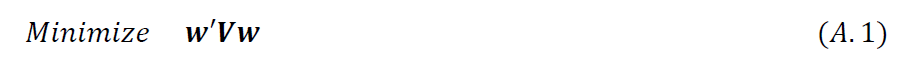subject to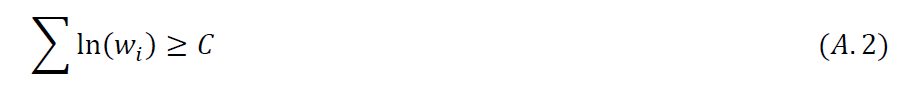where C is finite number. Clearly, the constraint (A.2) ensures that all the weights in the optimal portfolio are positive and non-zero.

The first order conditions for the solution of this problem are, together with (A.2):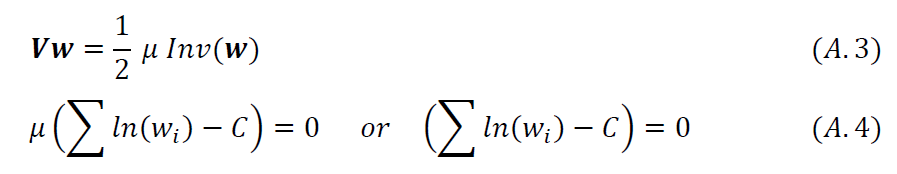where µ is the Lagrange multiplier associated with the constraint (A.2). Since V is positive definite, µ is necessarily larger than zero, and, therefore, the second of (A.4) follows from the first, which is the complementary slackness condition for the solution of (A.1) and (A.2).

It is straightforward to verify that if the pair {w*, λ*} is the solution of (5) and (6), the pair {w*, µ* = 2λ*} also solves (A.1) and (A.2), with C = ∑ln(w*i). Conversely, if the pair { 𝑤 , 𝜇 } is the solution of (A.1) and (A.2) for a specified value of C, the pair {𝜃𝑤 , 𝜃2𝜇 } solves this problem2 with θ = 1/∑wi and C = ∑ln(θw*i) and the pair {θw,1/2(θ2µ)} also solves the problem (5) and (6). Thus, risk parity portfolios are also minimum variance portfolios with a constraint that enforces the requirement that the weights of all the assets be nonzero and positive.

2 This property of the solution suggests that the parameter C of the problem is no longer arbitrary as soon as a normalization condition ∑wi = 1 is imposed on the solution w.